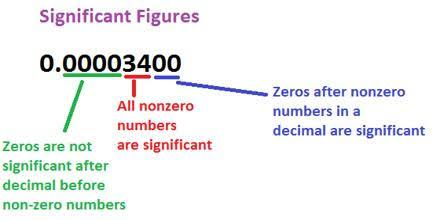# What Do You Know About Significant Figures?

10 Questions | Total Attempts: 346SettingsRegardless if you call them significant figures or significant digits, these are digits of a particular number, which carry meaning adding to its measurement resolution. They are a measurement that shows the number of important single digits in a value. Digits with all leading zeros, trailing zeros, or unauthentic digits are not significant figures.

• 1.
What is the first significant figure in 0.0010556?
• A.

1

• B.

0

• C.

5

• D.

6

• 2.
How many significant figures are in 101.1203?
• A.

4

• B.

5

• C.

6

• D.

7

• 3.
How many significant figures are in 0.00052?
• A.

2

• B.

5

• C.

6

• D.

3

• E.

1

• 4.
How many significant figures are in 12.2300?
• A.

6

• B.

4

• C.

2

• D.

3

• E.

1

• 5.
How many significant figures are in 0.000122300?
• A.

4

• B.

5

• C.

6

• D.

10

• 6.
What is the second significant figure in 0.0010123?
• A.

1

• B.

0

• C.

2

• D.

3

• 7.
How many significant figures are in 1025.5000?
• A.

4

• B.

8

• C.

5

• D.

7

• 8.
How do we round off 0.002546 to 3 significant figures?
• A.

0.002546

• B.

0.00255

• C.

0.00

• D.

0.00254

• 9.
What do we get when we round off 10605 to 2 significant figures?
• A.

106

• B.

11

• C.

10

• D.

1061

• 10.
Which of these is accurate for rounding off 0.01234500 to 7 significant figures?
• A.

0.01234500

• B.

0.01235000

• C.

0.0123450

• D.

0.0123500

Related TopicsBack to top